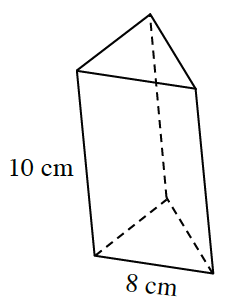### Home > CCG > Chapter 10 > Lesson 10.3.5 > Problem10-184

10-184.

Calculate the total surface area and volume of the prism below. The bases are equilateral triangles.

The equation for the volume of a prism is $\text{V = (area of base) · (height of prism)}$

Surface Area = $295.43$ square cm.
Volume = $277.13$ cubic cm.